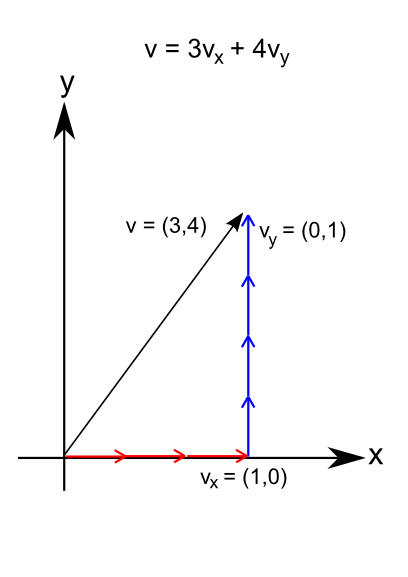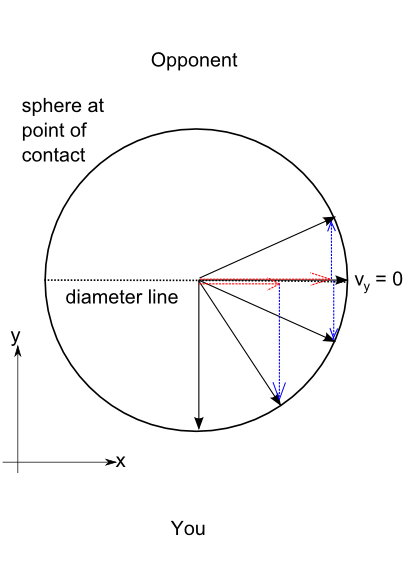## Kickin' Ass with Math: Vectors, spheres and points of contact

One of the topics that has come up in the past workshops is the idea of vector forces in the spheres of offense and defense.  To maintain your defense or invade your opponent's defense at the point of contact, the understanding of these spheres is essential.  The concept of the spheres can at first seem esoteric, but the forces involved can be understood with some elementary geometry and physics (warning, math to be discussed ahead).

The explanation will require revisiting vector math.  In case you fell asleep during the chapter on vector math, so we'll review it quickly.  To simplify things, we'll just explain things in two-dimensions.  A vector is just a magnitude (like distance, speed, amount of force, etc.) and a direction.  Vectors can be represented in a regular (x,y) Cartesian coordinate system.  Every vector can be expressed as a sum of other vectors, with the most convenient vectors being the x-vector vx and y-vector vy.  For example, a vector from the origin to point (3,4) is equivalent to the sum of 3*vx + 4*vy.Every point of contact can be understood as point on the outside of a sphere, or circle for purposes of illustration.  The diameter line divides the circle in half.  Every point on the circumference of the circle is a vector with an x and y component.  As we move along the circumference towards the diameter line, the x vector component gets bigger and the y vector component gets smaller.  When we hit the diameter line, there is no y component in the vector pointing from the center of the circle to the circumference point.  When we pass to the other side of the diameter line, the y-vector component switches sign (i.e. flips direction).What does this mean practically?  Remember that the point of contact is on the outside of a sphere.  The engagment force at the point of contact is the vector from the center of the sphere to the point of contact.  The importance of this is that when the point of contact reaches the diameter line, the force component preventing you from entering your opponent's space disappears (the y-vector goes to zero).  Once you pass the diameter line into your opponents' space, force components arise which help pull your attack into their space (i.e. the y-vector force points towards your opponent rather than at you).  Crossing the diameter line of the sphere is crossing to a point of contact which is "empty"; past the diameter line, you can enter with minimum effort and your opponent will find it difficult to engage you with enough force to stop you.

The theory is simple enough to understand, although not necessarily easy to put into practice.  To use the spheres to your advantage, you have to be aware enough to sense the spheres.  Then you must be able to flow along the circumference of the spheres to enter, while the point of contact is moving, the spheres are changing, and your opponent is trying to pass your defenses (penetrate your spheres).